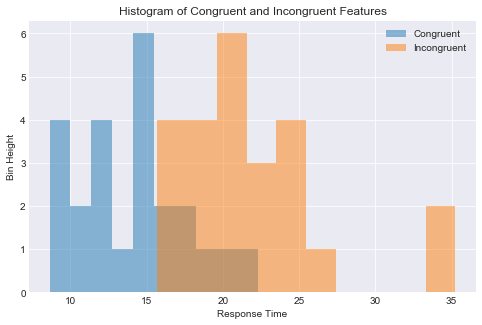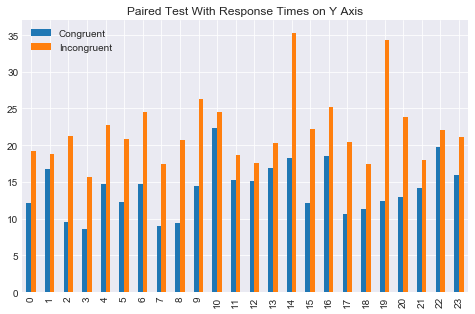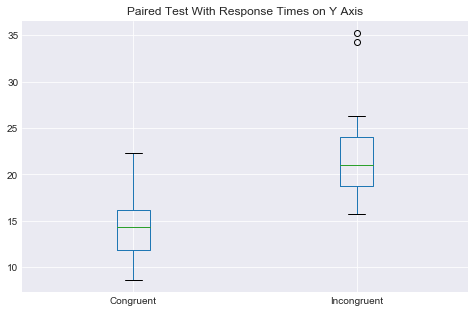### Analyzing the Stroop Effect¶

(1) What is the independent variable? What is the dependent variable?

In this dataset, we have taken control of the word/color congruency condition. The independent variable is whether the words shown are congruent with the ink colors or if they are incongruent with the ink colors. The dependent variable is 'Response Time'.

(2) What is an appropriate set of hypotheses for this task? Specify your null and alternative hypotheses, and clearly define any notation used. Justify your choices.

Even though there are a small number of rows in this dataset, we are treating this dataset as the entire population. As a result we are using μ as the population mean.

Symbol Explanation
$$H_0:$$ Null Hypothesis
$${μ}_{congruent}$$ The mean of the Congruent population's response times.
$${μ}_{incongruent}$$ The mean of the Incongruent population's response times.
$$H_1$$ Alternate Hypothesis

$$H_0: {μ}_{congruent} - {μ}_{incongruent} = 0$$

• The Null Hypothesis is: There is no difference between the population means of the two columns (Congruent and Incongruent) for response times. $$H_1: {μ}_{congruent} - {μ}_{incongruent} \neq 0$$
• The Alternative Hypothesis is: There is a difference between the population means of the two columns (Congruent and Incongruent) for response times..

We do not expect the Null Hypothesis to be true. This is the "negative" result. We do expect the mean of the response times for the incongruent columns to be higher. In keeping with standard statistical norms we will make the Null Hypothesis that the means are equal ("negative result"). This will be a two tailed test since the means will be tested diverging from each other in either direction (positive or negative).

The assumptions for these hypothesis are as follows:

• The data is true Stroop data.
• The values in the columns are response time data.
• This is the entire population (not just a sample) that we are dealing with.
• Each row is one individual test that is suitable for pairing (Congruent and Incongruent).

(3) Report some descriptive statistics regarding this dataset. Include at least one measure of central tendency and at least one measure of variability. The name of the data file is 'stroopdata.csv'.

In :
# Import packages
import pandas as pd
import scipy.stats as stats
stats.chisqprob = lambda chisq, df: stats.chi2.sf(chisq, df)
import matplotlib.pyplot as plt
%matplotlib inline
import seaborn as sns
sns.set_style('darkgrid')

In :
df = pd.read_csv('stroopdata.csv')

Out:
Congruent Incongruent
0 12.079 19.278
1 16.791 18.741
2 9.564 21.214
3 8.630 15.687
4 14.669 22.803

The pandas dataframe .describe method (below) produces the required descriptive statistics. We have the means, standard deviations, and the 5 number summaries (min, max, and quartiles). For central tendency we have the two means (14.0511 and 22.0159). For variability we have the standard deviations (3.5593 and 4.7971). As well for variability we also have the minimum and maximum for both columns.

In :
df.describe()

Out:
Congruent Incongruent
count 24.000000 24.000000
mean 14.051125 22.015917
std 3.559358 4.797057
min 8.630000 15.687000
25% 11.895250 18.716750
50% 14.356500 21.017500
75% 16.200750 24.051500
max 22.328000 35.255000
In :
# Observed difference between means
obs_diff = 22.0159 - 14.0511
obs_diff

Out:
7.9647999999999985

(4) Provide one or two visualizations that show the distribution of the sample data. Write one or two sentences noting what you observe about the plot or plots.

In :
# Build the visualizations here

In :
# Histograms for Congruent and Incongruent features
plt.figure(figsize=(8, 5))
plt.xlabel('Response Time')
plt.ylabel('Bin Height')
plt.hist(df['Congruent'], alpha=.5, label='Congruent')
plt.hist(df['Incongruent'], alpha=.5, label='Incongruent')
plt.title('Histogram of Congruent and Incongruent Features')
plt.legend();From the overlaid histograms you can clearly see that that the entire data set is shifted to the right for the Incogruent Feature. This means that on average the Incongruent Feature's response times are much larger than the Congruent Feature's response times. The histogram's apparent means are also consistent with the df.describe() method that we used above.

In :
df.plot(figsize=(8, 5), title='Paired Test With Response Times on Y Axis', kind='bar');This bar chart is even more instructive. For every test, it shows that the Incongruent Feature's response time is higher. There are NO exceptions.

In :
df.plot(figsize=(8, 5), title='Paired Test With Response Times on Y Axis', kind='box');The boxplot is also quite instructive. As you can see Incongruent's data range is consistently higher than all of the similiar values on Congruent (e.g. median, quartiles, min, max). There are two outliers on Incongruent, that are considerably larger than anything else in either Feature.

In :
df.sort_values('Incongruent')

Out:
Congruent Incongruent
3 8.630 15.687
7 8.987 17.394
18 11.344 17.425
12 15.073 17.510
21 14.233 17.960
11 15.298 18.644
1 16.791 18.741
0 12.079 19.278
13 16.929 20.330
17 10.639 20.429
8 9.401 20.762
5 12.238 20.878
23 16.004 21.157
2 9.564 21.214
22 19.710 22.058
15 12.130 22.158
4 14.669 22.803
20 12.944 23.894
10 22.328 24.524
6 14.692 24.572
16 18.495 25.139
9 14.480 26.282
19 12.369 34.288
14 18.200 35.255

(5) Now, perform the statistical test and report your results. What is your confidence level or Type I error associated with your test? What is your conclusion regarding the hypotheses you set up? Did the results match up with your expectations? Hint: Think about what is being measured on each individual, and what statistic best captures how an individual reacts in each environment.

• We will be using a paired T Test. From http://www.statstutor.ac.uk/resources/uploaded/paired-t-test.pdf This is used to compare two population means where you have two samples in which observations in one sample can be paired with observations in the other sample. This is exactly what we have here with the provided stroop data.
• We will be using a confidence level of .05 in a two tailed test.
• Conclusions will be provided below after we conduct the T Test.
In :
# Perform the statistical test here

In :
t, p = stats.ttest_rel(df.Congruent,df.Incongruent)
print('t-statistic =', t, 'p-value =', p)

t-statistic = -8.020706944109957 p-value = 4.103000585711178e-08

• From http://docs.statwing.com/examples-and-definitions/t-test/statistical-significance/ "A t-test’s statistical significance indicates whether or not the difference between two groups’ averages most likely reflects a “real” difference in the population from which the groups were sampled."
• A large t-statistic (and this is large) is typically accompanied by a statistically significant p-value. The p-value here is vanishingly small 4.103e-08 (ergo statistically significant).
• 4.103e-08 <.025 (our level of significance is .05 BUT this is a two tailed test). We would reject the Null Hypothesis and accept the Alternative Hypothesis. There is a signficant difference between the means of the two Features (Congruent and Incongruent).

(6) Optional: What do you think is responsible for the effects observed? Can you think of an alternative or similar task that would result in a similar effect? Some research about the problem will be helpful for thinking about these two questions!

• To make our lives simple, our brains are wired to simply respond to stimuli. We have learned over the years that most stimuli are congruent. When the wind blows the trees move. As a result, it is not necessary to measure the velocity of the wind. You can simply look out the window of the house and if the trees are moving, it is windy.
• When we encounter stimuli that is incongruent, all this pre-set up wiring needs neutralizing for us to properly respond. It takes some time to process the stimuli and respond correctly since we need to fight thru all the pre-conditioning that we have developed over a life time.
• The Stroop effect is all around us. An example would be a police lineup. Supposedly the perp is in the line up. It is congruent that the perp is there. So, the witness picks one of the people in the lineup. Yet, all that may be occurring is the Stroop effect. While this may sound academic, for the person that just got picked out of the lineup for a major crime, it is far from academic.
• The net is for a wide range of stimuli, there is built in congruency bias. This may be helpful for us to run our lives, but ... when true thinking is required, it is a large hurdle for us to a) recognize that is what is occurring and b) continue with the process to think rationally instead of reflexively.